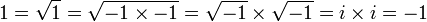## Another proof that -1=1

If you like this post, please share.

Here is a little maths teaser for you. Since I was a student, I always loved those “proofs” that zero equals one. Of course, most of the time, this was achieved by sneakily dividing by zero somewhere along the way.

But yesterday I came across a proof that used a different, slightly more subtle trick and uses complex numbers. I apologise to any reader not familiar with complex numbers. Anyone interested can find a quick introduction here.

Enough introduction, here is the “proof”:Looks OK, but it can’t be right of course. So where is the error in this equation? Can you find out?

Update:
You can find the answer here.

If you like this post, please share.

### 4 Responses

1.frosty840

It’s been a few years since A-level maths, but I think there are some brackets implied in the third stage, which then suffer some terrible fate in between the third and fourth stages…

2.Robert Lewis

This is an old one. The problem is that sqrt is not a function: every real and complex number has two square roots.

3.That depends on your definition of sqrt. If I define sqrt to be the principal square root then it is a function. But the problem above persists.

4.Bobby Thomas

The subtle error in the proof is the assumption that the property “sqrt(x * y) = sqrt(x) * sqrt(y)” holds for all real numbers. It is only valid for all x, y >= 0 where x, y are real numbers. The above “proof” can even be modified to yield an actual proof demonstrating that the property does not hold for ANY negative real numbers.